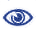# Prague Stringology Conference 2016

Algorithms to Compute the Lyndon Array

 Abstract: In the Lyndon array λ = λx[1..n] of a string x = x[1..n], λ[i] is the length of the longest Lyndon word starting at position i of x. The computation of λ has recently become of great interest, since it was shown (Bannai et al., The “Runs” Theorem) that the runs in x are computable in linear time from λx. Here we describe two algorithms for computing λx based on previous results known in different context, but for which no explicit exposition in this context had been given. These two algorithms execute in (n2) time in the worst case. The third algorithm presented that executes in Θ(n) time had been suggested and discussed previously, and we provide a more substantial discussion and prove of correctness for one of its steps. This algorithm achieves its linearity at the expense of prior computation of both the suffix array and the inverse suffix array of x. We then go on to sketch a new algorithm and its two variants that avoids prior computation of global data structures and indicate that in worst-case these algorithms perform in (n log n) time.

 Download paper:PostScript PDF BibTeX reference
 Download presentation: